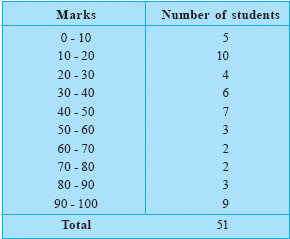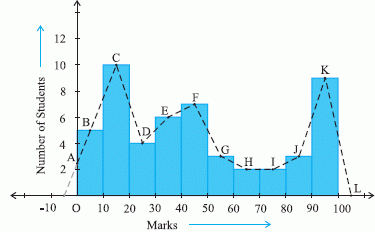Email us to get an instant 20% discount on highly effective K-12 Math & English kwizNET Programs!

#### Online Quiz (WorksheetABCD)

Questions Per Quiz = 2 4 6 8 10

### Middle/High School Algebra, Geometry, and Statistics (AGS)9.17 Frequency Polygon - 2

 Now let us consider a polygon where there is no class preceeding the first class. Let us take an example which gives us the marks scored by 50 students of a class, in an exam out of 100. The following table gives us the information about the data.Here the first class interval is 0 - 10. So the class preceeding it would lie on the negative X axis. The midpoint of the first class interval i.e. B is joined to this point with 0 frequency on the negative axis.Directions: On the basis of the given information answer the following questions
 Q 1: The points which represent the class marks of the class interval with the lowest frequency isH and IK and LA and BG and H Q 2: The difference between the highest and the lowest frequency is251028 Q 3: The cumulative frequency upto the class interval 30 - 40 is25354555 Q 4: Pt. A is the class mark of the class interval 0 - 10TrueFalse Question 5: This question is available to subscribers only! Question 6: This question is available to subscribers only!